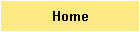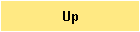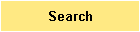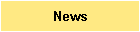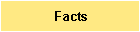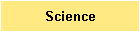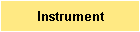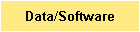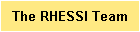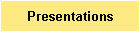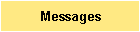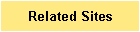Other RHESSI Web Sites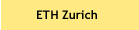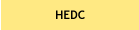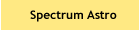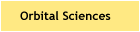# HESSI Demonstration Telescope

Introduction

The HESSI Demonstration Telescope is intended to show how HESSI will make images in hard x-rays and gamma rays.

The demonstration telescope will show that a pair of rotating grids modulates light from an off-axis point source.  Experimental data of the type in Boxes 3 and 4 in The HESSI Online Brochure are obtained with visible light.  Further experimentation is recommended to produce back projection patterns (Box 6 in The HESSI Online Brochure).

The demonstration telescope was designed by fifteen talented 4-8th grade students and mentored by Belinda Lowe-Schmahl, Ken Schmahl, Tom Mengel, and Dr. Ed Schmahl in the Schmahl Science Workshop in San Jose, California , .  Click here to link to their HESSI Telescope Demonstration web page.

Procedure

A point source of light is directed at the front of the demonstration telescope, at an angle from the telescope's axis of rotation.  Images are recorded at small angular increments over one revolution of the telescope.  These images show how the intensity of the light passing through the telescope varies as the telescope rotates.

The intensity of the light in the recorded images is plotted against roll angle.  It is expected that these plots can be approximated by the equation

P(a) = P0 + A cos(2pr sin(a-b)/p + L)  (Equation 1),

where P is the light intensity, a is the roll angle, P0 is the vertical offset in the plot, A is the amplitude of the oscillations in the plot, r is the offset angle of the light source, b is the initial roll angle of the telescope with respect to an arbitrary reference point, p is the angular pitch of the grids, and L is the phase difference between the front and rear grids.

Equation 1 indicates that the number of peaks in a graph of P(a) over one cycle should increase if r is increased or if p is decreased.

Apparatus

Click here for a description and photos of the demonstration telescope and equipment.

Results

Ten sets of images were recorded.  The first five sets were recorded using grids with a pitch of 0.90 cm; the remaining five sets were recorded using grids with a pitch of 0.59 cm.  In both sets the light source was at offset angles of 0.034, 0.048, 0.063, 0.095, and 0.161 radians.  Each set contains 176 images (two per mark on the telescope's rim - one at every mark, and one halfway between every two marks).  This corresponds to angular increments between images of about two degrees.

For each image, the average light intensity was calculated over an 80 pixel diameter disk in the center of the image (each image is 173 x 173 pixels).  The light intensity is the average pixel value, which ranges from 0 (black) to 255 (white).  The average light intensity is plotted against roll angle.

Below are plots of the ten sets of data.  Click on any plot to link to a page that contains a larger plot of the experimental data and a plot of Equation 1 with the appropriate values of P0, A, r, b, p, and L.  As the plots show, the number of peaks does indeed increase if r is increased or if p is decreased, as expected.

p = 9.0 mm                                          ;        p = 5.9 mm

r = 0.034 radians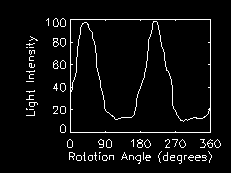r = 0.048 radians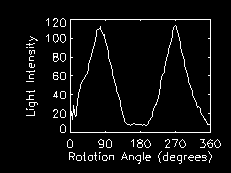r = 0.063 radians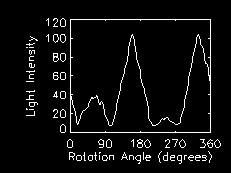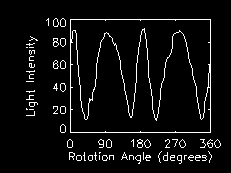r = 0.095 radians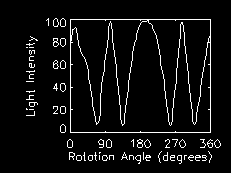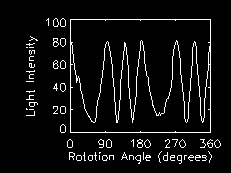r = 0.161 radians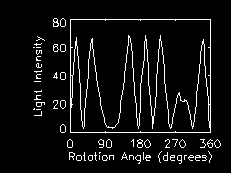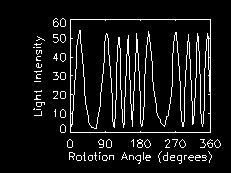A few notes on the determination of the parameters in Equation 1:

There is always some uncertainty involved in physical measurements.  In this experiment, uncertain measurements were made of r, p, and a; the other variables were determined by trial and error as they were adjusted to find the best fit to the plot of the experimental data.  The offset angle r was also determined in this manner, after it was first estimated with physical measurements.  These measurements were unreliable due to the difficulty of determining the location of the telescope's axis of rotation twenty feet away from the telescope.  All of the estimates of r were correct to within 0.02 radians - a difference that may have been caused by an error of about one degree in estimating the telescope's axis.

The uncertainties in measuring p and a are considerably smaller; the telescope and grids were measured with a ruler, and a was measured with the markings on the telescope's rim, which are fairly evenly spaced.  The values of p recorded, when plugged into Equation 1, and the values of a, when used with the experimental data, produced plots that were satisfactorily similar.

The angular pitch, p, is the angle subtended by one pitch of the front grids with respect to the detector (or in this case, the first layer of diffuser).  For all sets of data, the distance between front grid and diffuser was 9 cm; the angular pitches are therefore 0.9/9 = 0.1 and 0.59/9 = 0.07, approximately.

Ideally, the variable L should be the same for all sets of data taken using the same grids.  For modulation profiles with 0.90 cm pitch grids, L varies from 0.22 to 0.3 radians, except for the last profile, which has L = 0.7.  However, the telescope was disassembled and reassembled before this final set of data was taken, whereas the other four were obtained without any alteration of the telescope.  For modulation profiles with 0.59 cm pitch grids, L varies from 0 to 0.22 (there was no alteration of the telescope between any of these sets of data).  This is a much larger range than in the case of the first four of the profiles with the larger pitch; of course, the greater the pitch, the smaller the phase difference that corresponds to a specific distance.  A range of 0.22 radians corresponds to a shift of only about 0.2 mm with the 5.9 mm pitch grids.  This shift may be caused by the wobble of the telescope as it turns.

References

1. An Optical Demonstration Project For The HESSI Rotating Modulation Collimator
Bulletin of the American Astronomical Society, Vol. 32, No. 2, 2.122
2.

3. HESSI Telescope Demonstration
http://www.schmahlscience.org/awards.html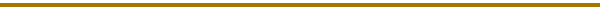NASA Goddard Space Flight Center Homepage

NASA Homepage

Sally House, summer intern, 2000
sallyh@wpi.edu#### Web Design:  Merrick Berg, Brian Dennis, Gordon Holman, & Gilbert Prevost

Heliophysics Science Division
NASA/Goddard Space Flight Center
Laboratory for Solar Physics/ Code 671
Greenbelt, MD, 20771, USA
Gordon.D.Holman@nasa.gov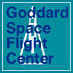+ NASA Privacy Statement, Disclaimer, and Accessibility CertificationThis site last updated November 10, 2008.